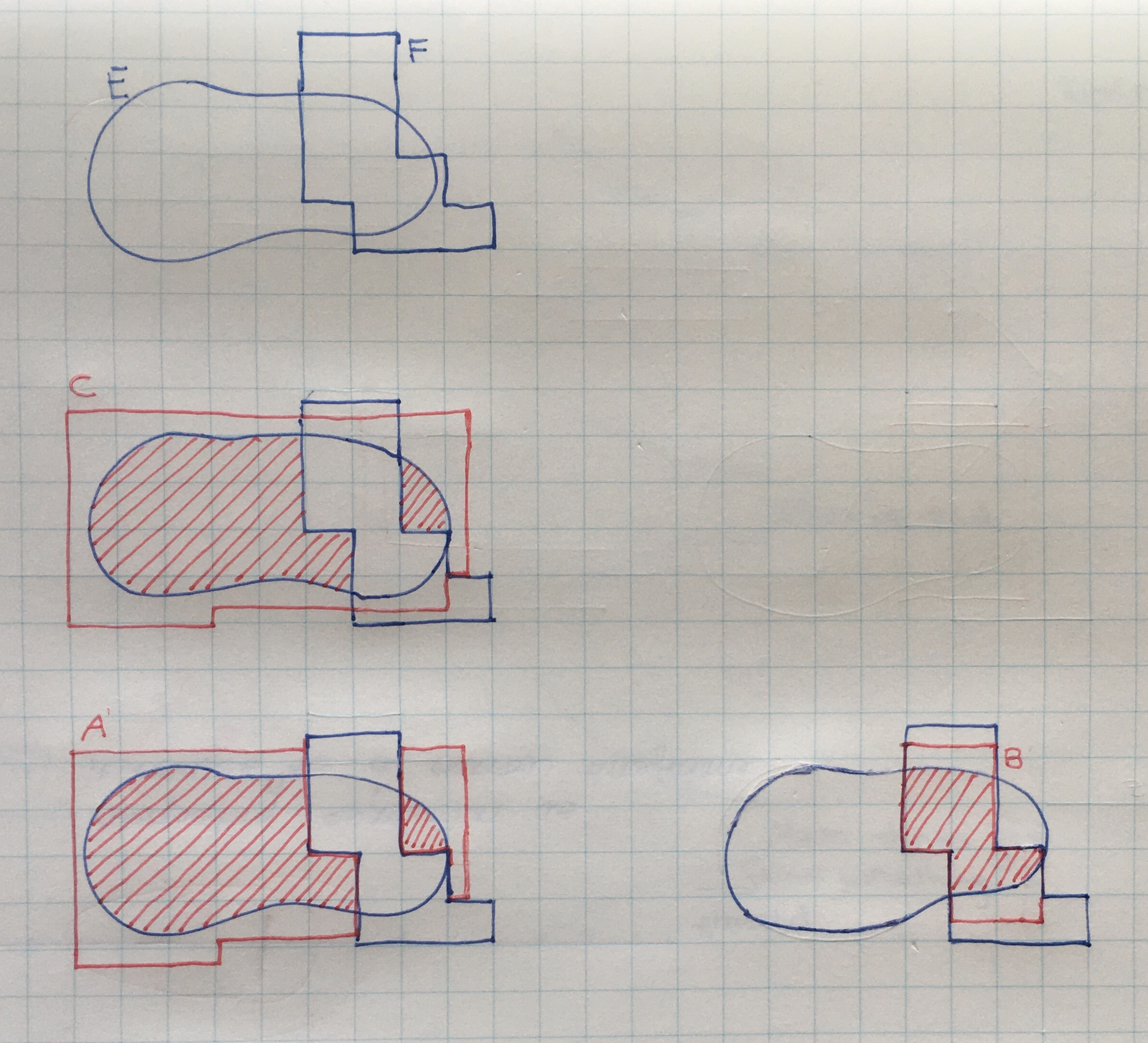Show Question
Math and science::Analysis::Tao, measure::01. Jordan measure

# Carathéodory type property

Let $$E \subset \mathbb{R}^d$$ be a bounded set, and let $$F \subset \mathbb{R}^d$$ be an elementary set. It is true that:

$m^{*,(J)}(E) = m^{*,(J)}(E \cap F) + m^{*,(J)}(E \setminus F)$

### Proof outline

An elementary cover $$C$$ of a set $$E$$ can be broken into two disjoint elementary sets, $$A$$ and $$B$$ which union to $$C$$. This can be done by taking the intersection and difference of $$C$$ with some other elementary set $$F$$. $$A$$ will be a cover for $$E \setminus F$$ and $$B$$ a cover for $$E \cap F$$. As $$A$$ and $$B$$ are disjoint, we have $$m(A \cup B) = m(A) + m(B)$$. $$A$$ and $$B$$ will have an elementary measure larger than the infimum representing $$m^{*,(J)}(E \setminus F)$$ and $$m^{*,(J)}(E \cap F)$$ respectively, however, they will have a summed measure smaller than that of $$C$$ which can be made arbitrarily close to $$m^{*,(J)}(E)$$.### Implication

In this formulation, the Carathéodory type property can be thought of as being one answer to the question: under what conditions do we have finite additivity of outer Jordan measure (not mere sub-additivity)?

This question is especially pertinent when considering the fact that Jordan outer measure, while additive for Jordan measurable sets, is not additive in general: the outer measure of the union of the bullets and the bullet-riddled square is 1 (as union is $$[0,1]$$), yet the outer measure of each set individually is also 1.

The answer is that if a set $$E$$ is split by an elementary set into $$A$$ and $$B$$, then any cover of $$E$$ can be split (without repeat) and cover $$A$$ and $$B$$. This makes $$m^{*,(J)}(A) + m^{*,(J)}(B) \le m^{*,(J)}(E)$$. The reverse inequlity is simply the characteristic of sub-additivity, which is indeed possessed by outer measure.

### Proof

For any real $$\delta > 0$$ there exists an elementary set $$C$$ covering $$E$$ with elementary measure $$m(C) \le m^{*,(J)}(E) + \delta$$. $$C$$ can be broken into two disjoint elementary sets $$A = C \setminus F$$ and $$B \cap F$$ for some elementary set $$F$$, and we will have $$m(C) = m(A) + m(B)$$. This means that $$m(C) = m(A) + m(B) \le m^{*,(J)}(E) + \delta$$. It is true (can be checked with some set algebra) that $$A$$ is a cover for $$E \setminus F$$ and $$B$$ a cover for $$E \cap F$$. Consequently, $$m(A) \ge m^{*,(J)}(E \setminus F)$$ and $$m(B) \ge m^{*,(J)}(E \cap F)$$. Substituting this back into the previous inequality we see that:

$m^{*,(J)}(E \setminus F) + m^{*,(J)}(E \cap F) \le m^{*,(J)}(E) + \delta$

$$\delta$$ was arbitrary so we can say:

$m^{*,(J)}(E \setminus F) + m^{*,(J)}(E \cap F) \le m^{*,(J)}(E)$

That proves the harder of the two inequalities needed! Paired with the sub-additivity of outer Jordan measure, we have equality:

$m^{*,(J)}(E \setminus F) + m^{*,(J)}(E \cap F) = m^{*,(J)}(E)$